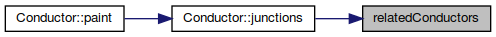QElectroTech  0.8-dev
conductor.h File Reference
`#include "conductorproperties.h"`
`#include <QGraphicsPathItem>`
`#include "assignvariables.h"`
Include dependency graph for conductor.h: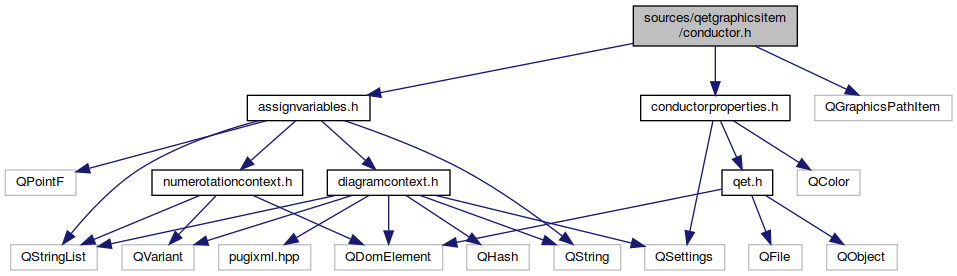This graph shows which files directly or indirectly include this file: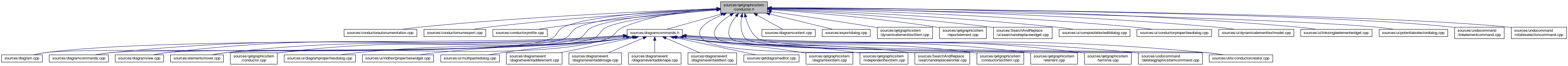Go to the source code of this file.

class  Conductor

## Typedefs

typedef QPair< QPointF, Qt::Corner > ConductorBend

typedef QHash< Qt::Corner, ConductorProfileConductorProfilesGroup

## Functions

ConductorlonguestConductorInPotential (Conductor *conductor, bool all_diagram=false)
longuestConductorInPotential More...

QList< Conductor * > relatedConductors (const Conductor *conductor)
relatedConductors More...

template<typename T >
bool isBetween (const T a, const T b, const T c)

## ◆ ConductorBend

 typedef QPair ConductorBend

## ◆ ConductorProfilesGroup

 typedef QHash ConductorProfilesGroup

## ◆ isBetween()

template<typename T >
 bool isBetween ( const T a, const T b, const T c )
Here is the caller graph for this function: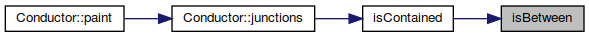## ◆ longuestConductorInPotential()

 Conductor* longuestConductorInPotential ( Conductor * conductor, bool all_diagram )

longuestConductorInPotential

Parameters
 conductor : a conductor in the potential to search all_diagram : true -> search in the whole project, false -> search only in the diagram of conductor
Returns
the longuest conductor in the same potential of conductor
Here is the call graph for this function: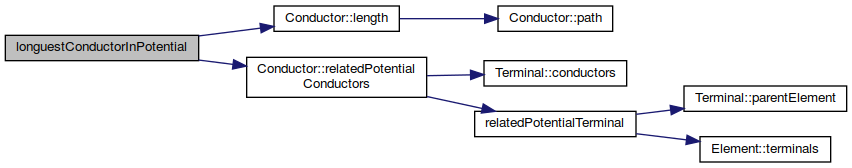Here is the caller graph for this function: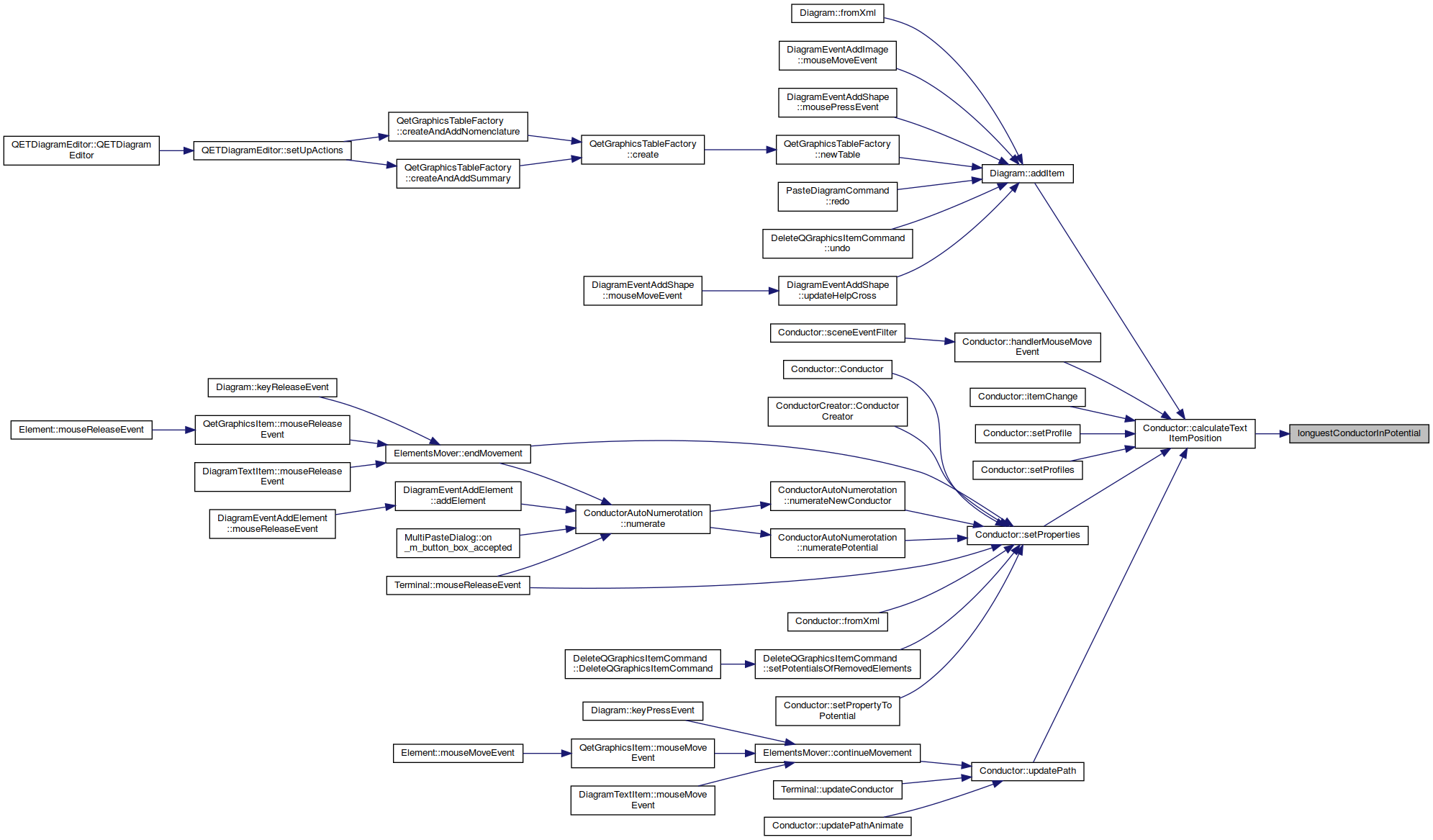## ◆ relatedConductors()

 QList relatedConductors ( const Conductor * conductor )

relatedConductors

Parameters
 conductor
Returns
return all conductors who share the same terminals of conductor given as parametre, except conductor himself.
Here is the call graph for this function: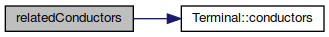Here is the caller graph for this function: